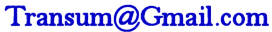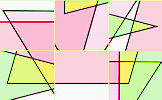# Factorising

## Factorise algebraic expressions in this structured online self marking exercise.

##### Level 1Level 2Level 3Level 4Level 5Level 6Level 7Level 8Level 9DescriptionHelpAlgebra

This is level 1: factorising an expression with a constant factor. You can earn a trophy if you get at least 9 questions correct and you do this activity online.

Factorise the following expressions. Order the terms in your answers in the same order as they appeared in the question.

 $$6a + 8$$$$15b + 6$$$$25c + 10$$$$35d + 10$$$$10e - 12$$$$44 + 20f$$$$21g - 33$$$$15h + 25$$$$44 - 28i$$$$10 + 12j + 34p$$$$55 - 25k - 85q$$$$22 + 10y + 82r$$Check

This is Factorising level 1. You can also try:
HCF Level 2 Level 3 Level 4 Level 5 Level 6 Level 7 Level 8 Level 9 More Quadratics Expanding Brackets More Algebra

## Instructions

Try your best to answer the questions above. Type your answers into the boxes provided leaving no spaces. As you work through the exercise regularly click the "check" button. If you have any wrong answers, do your best to do corrections but if there is anything you don't understand, please ask your teacher for help.

When you have got all of the questions correct you may want to print out this page and paste it into your exercise book. If you keep your work in an ePortfolio you could take a screen shot of your answers and paste that into your Maths file.

## Transum.org

This web site contains over a thousand free mathematical activities for teachers and pupils. Click here to go to the main page which links to all of the resources available.

Please contact me if you have any suggestions or questions.## More Activities:

Mathematicians are not the people who find Maths easy; they are the people who enjoy how mystifying, puzzling and hard it is. Are you a mathematician?

Comment recorded on the 26 March 'Starter of the Day' page by Julie Reakes, The English College, Dubai:

"It's great to have a starter that's timed and focuses the attention of everyone fully. I told them in advance I would do 10 then record their percentages."

Comment recorded on the 17 November 'Starter of the Day' page by Amy Thay, Coventry:

"Thank you so much for your wonderful site. I have so much material to use in class and inspire me to try something a little different more often. I am going to show my maths department your website and encourage them to use it too. How lovely that you have compiled such a great resource to help teachers and pupils.
Thanks again"

#### Polygon PiecesArrange the nine pieces of the puzzle on the grid to make the given polygon. Level one is for those learning the names of shapes while other levels are for those who like a challenge!

There are answers to this exercise but they are available in this space to teachers, tutors and parents who have logged in to their Transum subscription on this computer.

A Transum subscription unlocks the answers to the online exercises, quizzes and puzzles. It also provides the teacher with access to quality external links on each of the Transum Topic pages and the facility to add to the collection themselves.

Subscribers can manage class lists, lesson plans and assessment data in the Class Admin application and have access to reports of the Transum Trophies earned by class members.

If you would like to enjoy ad-free access to the thousands of Transum resources, receive our monthly newsletter, unlock the printable worksheets and see our Maths Lesson Finishers then sign up for a subscription now:

Subscribe

## Go Maths

Learning and understanding Mathematics, at every level, requires learner engagement. Mathematics is not a spectator sport. Sometimes traditional teaching fails to actively involve students. One way to address the problem is through the use of interactive activities and this web site provides many of those. The Go Maths page is an alphabetical list of free activities designed for students in Secondary/High school.

## Maths Map

Are you looking for something specific? An exercise to supplement the topic you are studying at school at the moment perhaps. Navigate using our Maths Map to find exercises, puzzles and Maths lesson starters grouped by topic.

## Teachers

If you found this activity useful don't forget to record it in your scheme of work or learning management system. The short URL, ready to be copied and pasted, is as follows:

Callum Arthur,

Tuesday, August 29, 2017

Do you have any comments? It is always useful to receive feedback and helps make this free resource even more useful for those learning Mathematics anywhere in the world. Click here to enter your comments.For All:

## Description of Levels

CloseLevel 1 - Factorising an expression with a constant factor.

Example: 40h + 88

Level 2 - Factorising an expression with a variable factor.

Example: 23h + 3h²

Level 3 - Factorising an expression with both a constant and variable factor.

Example: 10a + 2ab

Level 4 - Writing an expression of two parts that can be separately factorised.

Example: 3a + ab + 5w + 4wy

Level 5 - Writing an expression as the product of two binomials.

Example: 5a + 5b + ac + bc

Level 6 - Factorising an expression which is the difference between two squares.

Example: c² - 81

Level 7 - Factorising a quadratic where the squared term coefficient is 1.

Example: c² - 13c + 36

Level 8 - Factorising a quadratic where the squared term coefficient is not 1.

Example: 4a² + 5a - 6

Level 9 - Mixed factorisation questions

Example: b² + 6b + 9

Projectable large format factorising quadratics exercise suitable for whole class use.

Answers to this exercise are available lower down this page when you are logged in to your Transum account. If you don’t yet have a Transum subscription one can be very quickly set up if you are a teacher, tutor or parent.

## Examples

This program checks your answers by matching the text you have typed in with the options it has as the correct answer. For that reason it does not always recognise equivalent correct answers. For example the factorisation of 6a+21 can be written as 3(2a+7) or 3(7+2a) but the program may only recognise the first option as the correct answer. Please type in your answers so that the terms are in alphabetical order of the variables followed by the constants unless a negative cooeficient of the viariable makes the opposite a more elegant solution.

### Level 1 example: $$40h + 88$$

Identify a common factor of 8 to give $$8(5h+11)$$

### Level 2 example: $$23h + 3h²$$

Identify a common factor of h to give $$h(23+3h)$$

### Level 3 example: $$10a + 2ab$$

Identify a common factor of 2a to give $$2a(5+b)$$

### Level 4 example: $$3a + ab + 5w + 4wy$$

Identify common factors of pairs of terms to give $$a(3+b)+w(5+4y)$$

### Level 5 example: $$5a + 5b + ac + bc$$

Extract common factors of pairs of terms to give $$5(a+b) + c(a+b)$$
Then extract a common factor of (a+b) to give $$(a+b)(5+c)$$

### Level 6 example: $$c² - 81$$

This can be written as $$(c+9)(c-9)$$. Expand the brackets to see why

### Level 7 example: $$c² - 13c + 36$$

Start by finding two numbers with a product of 36 and sum of -13
These numbers are -4 and -9
So the factorisation is $$(c-4)(c-9)$$

### Level 8 example: $$4a² + 5a - 6$$

Multiply the coefficient of a² by the constant term: $$4 \times -6 = -24$$
Now find two numbers with a product of -24 and sum of 5 (the coefficient of a)
These numbers are -3 and 8
Write the original expression with the middle term split into these two numbers: $$4a^2-3a+8a-6$$
Extract common factors of pairs of terms to give $$a(4a-3) + 2(4a-3)$$
Then extract a common factor of (4a-3) to give $$(a+2)(4a-3)$$

### Level 9 example: $$b² + 6b + 9$$

Use the techniques used in the previous levels

Don't wait until you have finished the exercise before you click on the 'Check' button. Click it often as you work through the questions to see if you are answering them correctly. You can double-click the 'Check' button to make it float at the bottom of your screen.

Answers to this exercise are available lower down this page when you are logged in to your Transum account. If you don’t yet have a Transum subscription one can be very quickly set up if you are a teacher, tutor or parent.

Close